Question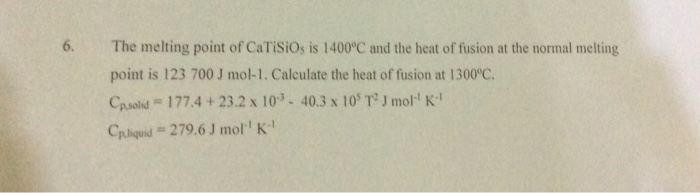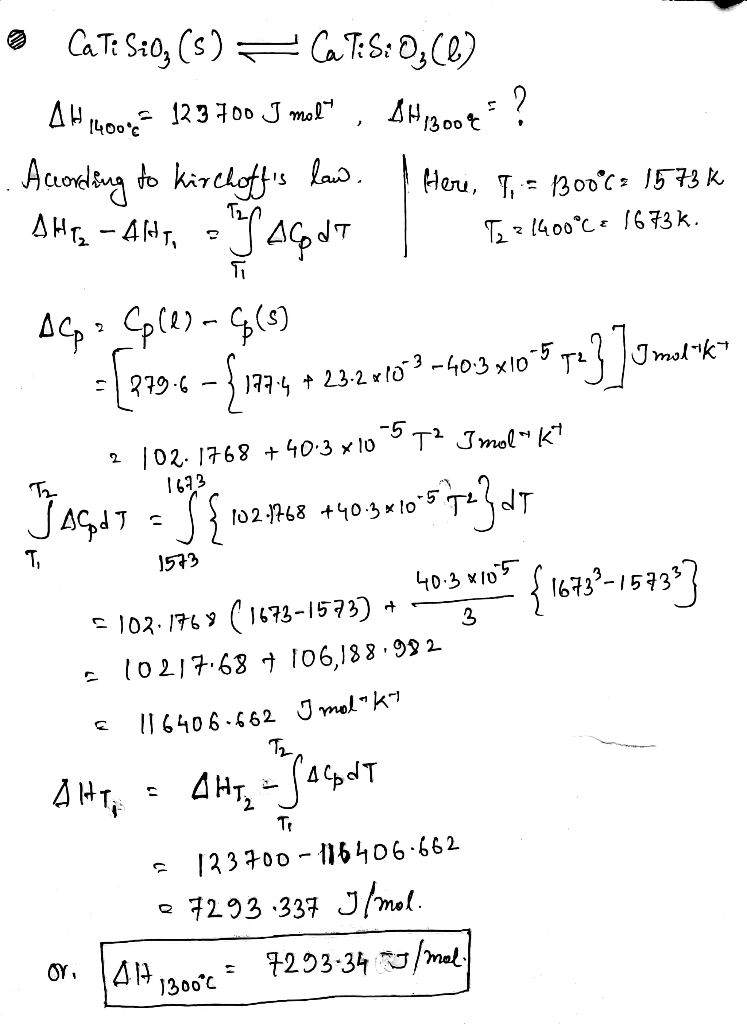#### Earn Coins

Coins can be redeemed for fabulous gifts.

Similar Homework Help Questions
• ### For benzene, C6H6, the heat of fusion at its normal melting point of 6 °C is...

For benzene, C6H6, the heat of fusion at its normal melting point of 6 °C is 10.0 kJ/mol. The entropy change when 1.86 moles of liquid C6H6 freezes at 6 °C, 1 atm is ? J/K.

• ### 14. For bismuth, Bi, the heat of fusion at its normal melting point of 271 °C...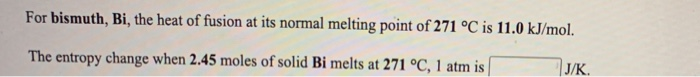14. For bismuth, Bi, the heat of fusion at its normal melting point of 271 °C is 11.0 kJ/mol. The entropy change when 2.45 moles of solid Bi melts at 271 °C, 1 atm is J/K. For magnesium, Mg, the heat of fusion at its normal melting point of 649 °C is 9.0 kJ/mol. The entropy change when 1.72 moles of liquid Mg freezes at 649 °C, 1 atm is J/K

• ### For ethanol, C2H5OH, the heat of fusion at its normal melting point of -115 °C is...

For ethanol, C2H5OH, the heat of fusion at its normal melting point of -115 °C is 5.0 kJ/mol. The entropy change when 1.84 moles of solid C2H5OH melts at -115 °C, 1 atm is J/K.

• ### For copper, Cu, the heat of fusion at its normal melting point of 1083 °C is...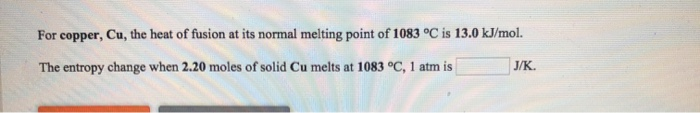For copper, Cu, the heat of fusion at its normal melting point of 1083 °C is 13.0 kJ/mol. The entropy change when 2.20 moles of solid Cu melts at 1083 °C, 1 atm is J/K

• ### The heat of fusion of toluene is 6.636×103J⋅mol−1 at its normal melting point of 178.15 K....

The heat of fusion of toluene is 6.636×103J⋅mol−1 at its normal melting point of 178.15 K. Calculate the freezing point depression constant Kf.

• ### The enthalpy of fusion of cadmium at its normal melting point of 321 °C is 6.11...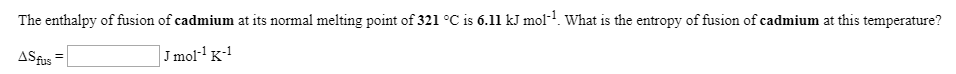The enthalpy of fusion of cadmium at its normal melting point of 321 °C is 6.11 kJ mol? What is the entropy of fusion of cadmium at this temperature? ASfus = J mol-K-1 The molar enthalpy of fusion of solid cadmium is 6.11 kJ mol-1, and the molar entropy of fusion is 10.3 JK+mol-1. (a) Calculate the Gibbs free energy change for the melting of 1.00 mol of cadmium at 622 K. (b) Calculate the Gibbs free energy change for...

• ### The enthalpy of fusion of bismuth at its normal melting point of 271 °C is 11.0...

The enthalpy of fusion of bismuth at its normal melting point of 271 °C is 11.0 kJ mol-1. What is the entropy of fusion of bismuth at this temperature? ΔSfus =  J mol-1 K-1

• ### Please answer clearly & correctly. Selected properties of water Heat of fusion at the normal melting...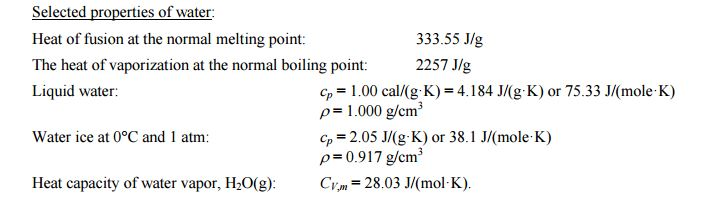Please answer clearly & correctly. Selected properties of water Heat of fusion at the normal melting point: The heat of vaporization at the normal boiling point: Liquid water: 333.55 J/g 2257 J/g Cp = 1.00 cal/(g. K) = 4.184 J/(g. K) or 75.33 J/(mole. K) ρ= 1 .000 g/cm3 Cp = 2.05 J/(g. K) or 38, l J/(mole K) ρ=0.917 g/cm3 Water ice at 0°C and 1 atm Heat capacity of water vapor, H20(g) Cvm 28.03 J/(mol-K).

• ### 18. Gallium metal has a heat of fusion, Hlusion, equal to 5.59 kJ/mol and a normal...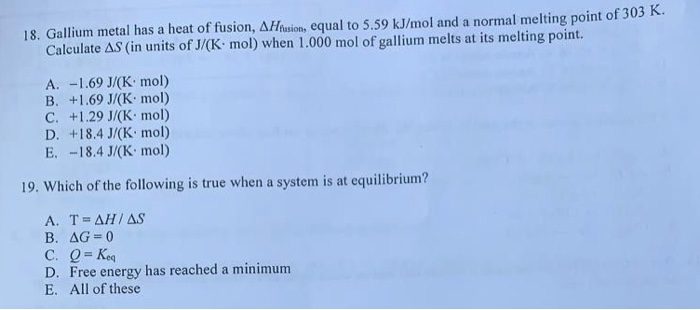18. Gallium metal has a heat of fusion, Hlusion, equal to 5.59 kJ/mol and a normal melting point of 305 m. Calculate AS (in units of J/(K mol) when 1.000 mol of gallium melts at its melting point. A. -1.69 J/(K: mol) B. +1.69 J/(K: mol) C. +1.29 J/(Kmol) D. +18.4 J/(Kmol) E. -18.4 J/(K mol) 19. Which of the following is true when a system is at equilibrium? A. T = AH/AS B. AG=0 C. Q = keq D....

• ### The change in enthalpy when 1 mol of ice is melted at 273K is 6008 J Heat Capacity of liquid water, Cp_{L} = 75.44 J/mol K Heat Capacity of solid water, Cp_{S} = 38J/mol K Enthalpy chage of melting at...

The change in enthalpy when 1 mol of ice is melted at 273K is 6008 J Heat Capacity of liquid water, Cp_{L} = 75.44 J/mol K Heat Capacity of solid water, Cp_{S} = 38J/mol K Enthalpy chage of melting at 273K, \Delta H_{273}=6008 J Calculate the standard enthalpy of fusion for ice. Calculate the heat released when 100 g of water supercooled at 250K solidify Initial T=25°C=298K Thanks